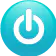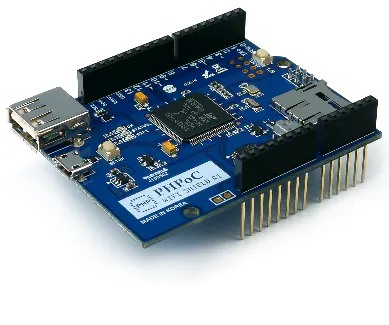# Arduino Dynamic Web Control

This project shows how to control a servo motor from a web browser in real -time using WebSocket.

BeginnerFull instructions provided4 hours54,532

## Things used in this project

### Hardware componentsArduino UNO
×1PHPoC WiFi Shield for Arduino
×1
 Servo motor
×1
 Jumper wires
×1

## Code

### remote_rotate.php

PHP
```<!DOCTYPE html>
<html>
<title>PHPoC Shield - Web Remote Control for Arduino</title>
<meta name="viewport" content="width=device-width, initial-scale=0.7, maximum-scale=0.7">
<style>
body { text-align: center; font-size: 15pt; }
h1 { font-weight: bold; font-size: 25pt; }
h2 { font-weight: bold; font-size: 15pt; }
button { font-weight: bold; font-size: 15pt; }
</style>
<script>
var canvas_width = 400, canvas_height = 400;
var click_state = 0;
var last_plate_angle = 0
var plate_angle = 0, last_angle = 0;
var ws;
function init()
{
var canvas = document.getElementById("remote");

var ctx = canvas.getContext("2d");

ctx.translate(canvas_width / 2, canvas_height / 2);

rotate_plate(0);
}
function connect_onclick()
{
if(ws == null)
{
var debug = document.getElementById("debug");
if((navigator.platform.indexOf("Win") != -1) && (ws_host_addr.charAt(0) == "["))
{
// network resource identifier to UNC path name conversion
}
//debug.innerHTML = "<br>" + navigator.platform + " " + ws_host_addr;
ws = new WebSocket("ws://" + ws_host_addr + "/remote_slide", "text.phpoc");
document.getElementById("ws_state").innerHTML = "CONNECTING";
ws.onopen = ws_onopen;
ws.onclose = ws_onclose;
ws.onmessage = ws_onmessage;
}
else
ws.close();
}
function ws_onopen()
{
document.getElementById("ws_state").innerHTML = "<font color='blue'>CONNECTED</font>";
document.getElementById("bt_connect").innerHTML = "Disconnect";
}
function ws_onclose()
{
document.getElementById("ws_state").innerHTML = "<font color='gray'>CLOSED</font>";
document.getElementById("bt_connect").innerHTML = "Connect";
ws.onopen = null;
ws.onclose = null;
ws.onmessage = null;
ws = null;
}
function ws_onmessage(e_msg)
{
e_msg = e_msg || window.event; // MessageEvent
}

function rotate_plate(angle)
{
var canvas = document.getElementById("remote");
var ctx = canvas.getContext("2d");

ctx.clearRect(-canvas_width / 2, -canvas_height / 2, canvas_width, canvas_height);
ctx.rotate(angle / 180 * Math.PI);

ctx.strokeStyle="red";
ctx.fillStyle="red";
ctx.beginPath();
ctx.arc(0, 0, plate_radius, Math.PI, Math.PI * 1.5);
ctx.lineTo(0, 0);
ctx.fill();
ctx.stroke();

ctx.strokeStyle="blue";
ctx.fillStyle="blue";
ctx.beginPath();
ctx.arc(0, 0, plate_radius, 0, Math.PI / 2);
ctx.lineTo(0, 0);
ctx.fill();
ctx.stroke();

ctx.strokeStyle="black";
ctx.arc(0, 0, plate_radius, 0, Math.PI * 2);
ctx.stroke();

ctx.rotate(-angle / 180 * Math.PI);
}
function mouse_down()
{
if(ws == null)
return;

var dist_min, dist_max, dist;

event.preventDefault();

if(event.offsetX)
{
x = event.offsetX - canvas_width / 2;
y = canvas_height / 2 - event.offsetY;
dist_min = 40;
}
else if(event.layerX)
{
x = event.layerX - canvas_width / 2;
y = canvas_height / 2 - event.layerY;
dist_min = 40;
dist_max = canvas_width / 2;
}
else
{
x = (Math.round(event.touches.pageX - event.touches.target.offsetLeft)) - canvas_width / 2;
y = canvas_height / 2 - (Math.round(event.touches.pageY - event.touches.target.offsetTop));
dist_min = 40;
dist_max = canvas_width / 2;
}

var dist = Math.round(Math.sqrt(x * x + y * y));
if((dist > dist_min) && (dist < dist_max))
{
click_state = 1;
last_angle = Math.round(Math.atan2(y, x) / Math.PI * 180);
}
}
function mouse_up()
{
if(ws == null)
return;

event.preventDefault();

click_state = 0;
}
function mouse_move()
{
if(ws == null)
return;

var dist_min, dist_max, angle, dist;

event.preventDefault();

if(!click_state)
return;

if(event.offsetX)
{
x = event.offsetX - canvas_width / 2;
y = canvas_height / 2 - event.offsetY;
dist_min = 40;
}
else if(event.layerX)
{
x = event.layerX - canvas_width / 2;
y = canvas_height / 2 - event.layerY;
dist_min = 40;
dist_max = canvas_width / 2;
}
else
{
x = (Math.round(event.touches.pageX - event.touches.target.offsetLeft)) - canvas_width / 2;
y = canvas_height / 2 - (Math.round(event.touches.pageY - event.touches.target.offsetTop));
dist_min = 40;
dist_max = canvas_width / 2;
}

angle = Math.round(Math.atan2(y, x) / Math.PI * 180);
dist = Math.round(Math.sqrt(x * x + y * y));

if((dist < dist_min) || (dist > dist_max))
{
click_state = 0;
return;
}

if((Math.abs(angle) > 90) && (angle * last_angle < 0))
{
if(last_angle > 0)
last_angle = -180;
else
last_angle = 180;
}

plate_angle += (angle - last_angle);
last_angle = angle;

if(plate_angle > 180)
plate_angle = 180;

if(plate_angle < 0)
plate_angle = 0;

rotate_plate(-plate_angle);

if(plate_angle != last_plate_angle)
{
{
ws.send("C" + plate_angle.toString() + "\r\n");
last_plate_angle = plate_angle;
debug = document.getElementById("debug");
debug.innerHTML = plate_angle;
//console.log(plate_angle);
}
}
}
</script>

<body>

<p>
<h1>Web Remote Control / Rotate</h1>
</p>

<canvas id="remote" width="400" height="400"></canvas>

<h2>
<p>
WebSocket : <span id="ws_state">null</span><br>
Angle : <span id="debug">0</span>
</p>
<button id="bt_connect" type="button" onclick="connect_onclick();">Connect</button>
</h2>

</body>
</html>
```

### Arduino code

Arduino
```/* arduino web server - remote control (slide switch) */

#include "SPI.h"
#include "Phpoc.h"
#include <Servo.h>

PhpocServer server(80);
Servo myservo;  // create servo object to control a servo

char slideName;
int slideValue;

void setup() {
Serial.begin(9600);
while(!Serial)
;

Phpoc.begin(PF_LOG_SPI | PF_LOG_NET);
//Phpoc.begin();

server.beginWebSocket("remote_slide");

Serial.println(Phpoc.localIP());

myservo.attach(9);  // attaches the servo on pin 9 to the servo object
myservo.write(1);
}

void loop() {
// wait for a new client:
PhpocClient client = server.available();

if (client) {

if(slideStr)
{
slideValue = slideStr.substring(1).toInt();
myservo.write(slideValue);
Serial.print("Angle: ");
Serial.println(slideValue);
}
}
}
```

## Credits

### phpoc_man

62 projects • 346 followers